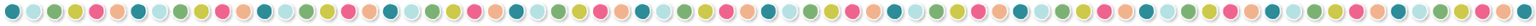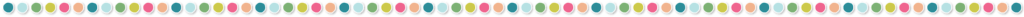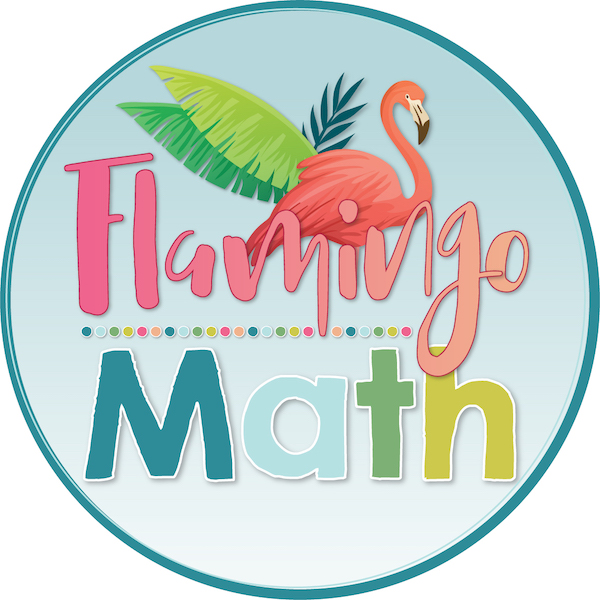# 4 Big Theorems About Polynomials

### Four Big Theorems About Polynomials

Algebra 2 is filled with so many rich topics and concepts to be discovered. I want to take a look at Polynomial Functions today.  We establish vocabulary terms and review factoring methods from Algebra 1, then it’s time to explore end-behavior, multiple roots and function behavior, relative extrema, and theorems about polynomials.  But first, our students need some new computational skills, long division and synthetic division.  Both are valuable techniques and need to be practiced for future classes.  (I’m thinking about simplifying improper integrals for Calculus in the future.)

### 1) The Remainder Theorem (Division Algorithm)

Let’s revisit 5th grade math! The dividend is equal to the quotient times the divisor plus the remainder. I always start with a simple numeric example with my students. Be patient! Remind them that it’s been a long time since they were in the 5th grade, so they need to be patient, too.  For example, 163 divided by 3 is 54 with a remainder of 1.
Using the theorem 163=(54)(3)+1. Got it! Piece of cake!

You know the routine, we teach polynomial long division. Now, let’s show them a short cut using synthetic division. (Did you see any mutiny? Remind them that there will be cases when only long division will work!) Then, move on to using the other theorems. NO!!!! Wait, there is rich material we are leaving out here. Take some time to look at the graphical results of these algebraic skills. Be sure students have ample time to discover that direct substitution of a value or synthetic substitution of the same value leads to a y-value or ordered pair on the graph of the function.

#### Spend some time at this beginning stage to ask those value rich questions.

“When the remainder is zero, what happens on the graph of the polynomial?”
“When the remainder is any number other than zero, what does that tell you about the polynomial?”

### 2) The Factor Theorem

The factor theorem basically defines the vocabulary of a factor and a zero of the function. That is, (x-a) is a factor of some polynomial P(x) if and only if P(a) = 0.  Will students see that when x = a, they have found a solution of the polynomial? Consider the example at right,

What are the factors of this polynomial?”
“What would the divisor look like to have no remainder (a solution) in the division algorithm?”
“Can you have a remainder of 3?” How?

### 3) The Rational Root Theorem

If you spend sufficient time with the first theorem, the Rational Root Theorem can be discovered intuitively. You can lead your students, by inquiry, to stumble upon the p/q rule when the leading coefficient is 1 through observations and questions.
What happens when the leading coefficient is 1?”
Can you find a rule for finding zeros?”
“How is the constant term helpful?”

Consider the following two functions:

### 4) The Complex Conjugate Theorem

This theorem brings out the big guns for our students! I prefer to introduce the Conjugate Root Theorem first, to give insight into the idea that irrational roots occur in conjugate pairs. Remind students that they have done this in Algebra 1 when solving quadratic functions by using the square root method. This will reinforce the computational reason that students need to add both positive and negative roots. Hopefully, the light bulb goes off and the connection to why there are two square roots for every non-negative number.  Also…that these two solutions…are now called conjugate pairs! YEAH!

Then they will be prepared to explore the conjugate pairs of complex numbers:
(a + bi) and (a – bi).

You’ll want to get this FREE GRAPHIC ORGANIZER for Theorems About Polynomials here!

Subscribe to our mailing list to receive a FREE GNAW ACTIVITY for Theorems About Polynomials.

### Subscribe to our mailing list!

We will send you the FREE GNAW ON POLYNOMIALS ACTIVITY